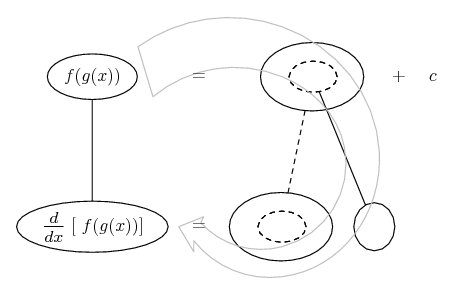# Thread: Chain rule/Derivative Calculation

1. ## Chain rule/Derivative Calculation

Hi,
Would anyone be able to tell me how I'd go about solving a problem like this. Are you taking the derivative of the entire problem or just the one in the parenthesis (f(4x^3))? The inclusion of (f) is also throwing me off, not sure if that's supposed to be part of the solution. Is the answer supposed be something like = 12x^2(f'(4x^3))? Any help on this would be greatly appreciated. Thanks! Ana

Here's the problem . . .

d/dx(f(4x^3)) = 6x^5

Calculate f'(x) = ?

2. ## Re: Chain rule/Derivative Calculation

We are given:

$\displaystyle \frac{d}{dx}\left(f\left(4x^3 \right) \right)=6x^5$

Using the chain rule, we find:

$\displaystyle f'\left(4x^3 \right)\cdot12x^2=6x^5$

$\displaystyle f'\left(4x^3 \right)=\frac{x^3}{2}=\frac{4x^3}{8}$ hence:

$\displaystyle f'(x)=\frac{x}{8}$

3. ## Re: Chain rule/Derivative Calculation

Just in case a picture helps...... where (key in spoiler) ...

Spoiler:... is the chain rule. Straight continuous lines differentiate downwards (integrate up) with respect to the main variable (in this case x), and the straight dashed line similarly but with respect to the dashed balloon expression (the inner function of the composite which is subject to the chain rule).

The general drift is..._________________________________________

Don't integrate - balloontegrate!

Balloon Calculus; standard integrals, derivatives and methods

Balloon Calculus Drawing with LaTeX and Asymptote!

4. ## Re: Chain rule/Derivative Calculation

I've been trying to figure this problem out for about an hour now. Thanks so much for breaking it down step by step; and for the picture!

5. ## Re: Chain rule/Derivative Calculation

The question is related to single derivative function. solving it as:
(d(f(4x^3)/dx)=6x^5
=>3*4x^2.f'(4x^3)=6x^5
f('4x^3)=6x^5/12x^2
=>f'(4x^3)=x^3/2
=>f'(x)=x^3/(2*4x^2)
=>f'(x)=x/8

Derivative is defined as a measure of how a function changes as its input changes. This process of finding derivative is called differentiation.

#### Search Tags

calculation, chain, rule or derivative